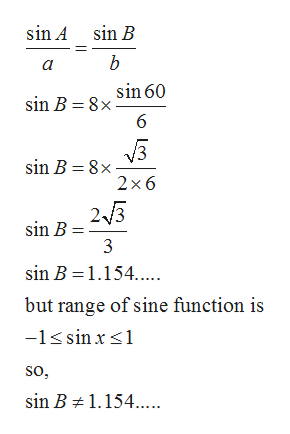# Triangle ABC has side a, b, c, opposite angles A, B, C, respectively. Find the request parts of the triangle or indicate if no triangle is possible. If more than one triangle is possible, find all possible solutions. Calculate all solutions to the nearest tenth. Angles are in degrees. a=6inb=8inA=60 degrees.Find B

Question

Triangle ABC has side a, b, c, opposite angles A, B, C, respectively. Find the request parts of the triangle or indicate if no triangle is possible. If more than one triangle is possible, find all possible solutions. Calculate all solutions to the nearest tenth. Angles are in degrees.

a=6in

b=8in

A=60 degrees.

Find B

check_circleExpert Solution
Step 1

Given figure is

Step 2

Now, calculating the angle B...help_outlineImage Transcriptionclosesin A sin B b a sin 60 sin B 8x 6 sin B 8x 2 x 6 25 sin B 3 sin B 1.154... but range of sine function is -1sin x so, sin B 1.154. fullscreen

### Want to see the full answer?

See Solution

#### Want to see this answer and more?

Solutions are written by subject experts who are available 24/7. Questions are typically answered within 1 hour*

See Solution
*Response times may vary by subject and question
Tagged in

### Other